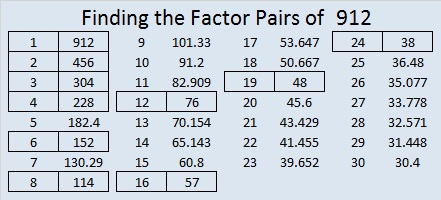# 912 and Level 6

912 is the sum of the ten prime numbers from 71 to 109.

It is also the sum of these four consecutive primes:

• 223 + 227 + 229 + 233 = 912

912 is 192 in BASE 26 because 1(26²) + 9(26) + 2(1) = 912.Print the puzzles or type the solution on this excel file: 12 factors 905-913

• 912 is a composite number.
• Prime factorization: 912 = 2 × 2 × 2 × 2 × 3 × 19, which can be written 912 = 2⁴ × 3 × 19
• The exponents in the prime factorization are 4, 1 and 1. Adding one to each and multiplying we get (4 + 1)(1 + 1)(1 + 1) = 5 × 2 × 2 = 20. Therefore 912 has exactly 20 factors.
• Factors of 912: 1, 2, 3, 4, 6, 8, 12, 16, 19, 24, 38, 48, 57, 76, 114, 152, 228, 304, 456, 912
• Factor pairs: 912 = 1 × 912, 2 × 456, 3 × 304, 4 × 228, 6 × 152, 8 × 114, 12 × 76, 16 × 57, 19 × 48 or 24 × 38
• Taking the factor pair with the largest square number factor, we get √912 = (√16)(√57) = 4√57 ≈ 30.1993377.This site uses Akismet to reduce spam. Learn how your comment data is processed.